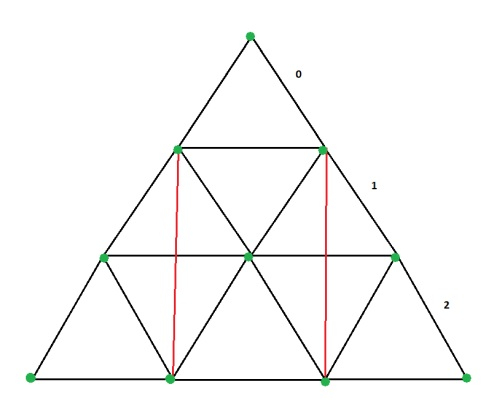# Count of distinct rectangles inscribed in an equilateral triangle in C++

We are an equilateral triangle with side length. The goal is to count the number of distinct rectangles that can be present inside the triangle such that horizontal sides of the rectangle are parallel to the base. Also all end points of the rectangle touch the dots as shown.Let us understand with examples

Input − sides=3

Output − Count of distinct rectangles inscribed in an equilateral triangle are − 1

Explanation − The figure above shows the rectangle.

Input − sides=10

Output − Count of distinct rectangles inscribed in an equilateral triangle are − 200

## Approach used in the below program is as follows

As from the above figure it is seen that horizontal edges will exist between dots of alternate levels.

The number of dots can be counted from level 0-1, level 1-2 ... level n-n+1.

• Input the side as an integer variable and pass it to function for further processing

• Take temporary variables as count, temp and check.

• Check IF sides are odd then start loop FOR i to sides - 1 till i greater than 1

• Inside the loop, check IF i & 1 then set temp as (sides - i)/2 and set check as (i * (i + 1))/2 and set count as check * temp ELSE, set temp as ((sides - 1) - i)/2 and also set check as (i * ( i + 1))/2 and set count as check * temp

• Else, if sides are even then start another loop FOR with i to be sides - 1 and i greater than 1.

• Inside the loop, check IF i & 1 then set temp as ((sides - 1) - i ) / 2 and set check as (i * (i + 1)) / 2 and set count as check * temp ELSE set temp as (sides - i) / 2 and set check as (i * (i + 1)) / 2 and set count as check * temp.

• Return count

• Print the result.

## Example

Live Demo

#include <iostream>
using namespace std;
int rec_inside_equi(int sides){
int count = 0, temp, check;
if(sides%2 != 0){
for(int i = sides - 2; i >= 1; i--){
if (i & 1){
temp = (sides - i) / 2;
check = (i * (i + 1)) / 2;
count += check * temp;
}
else{
temp = ((sides - 1) - i) / 2;
check = (i * (i + 1)) / 2;
count += check * temp;
}
}
}
else{
for(int i = sides - 2; i >= 1; i--){
if (i & 1){
temp = ((sides - 1) - i) / 2;
check = (i * (i + 1)) / 2;
count += check * temp;
}
else{
temp = (sides - i) / 2;
check = (i * (i + 1)) / 2;
count += check * temp;
}
}
}
return count;
}
int main(){
int sides = 4;
cout<<"Count of distinct rectangles inscribed in an equilateral triangle are: "<<rec_inside_equi(sides);
return 0;
}

## Output

If we run the above code it will generate the following output −

Count of distinct rectangles inscribed in an equilateral triangle are: 4